# Area of Plane Shapes

Area is the size of a surface!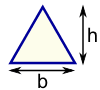Triangle Area = ½ × b × h b = base h = vertical height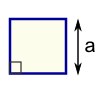Square Area = a2 a = length of side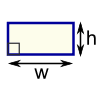Rectangle Area = w × h w = width h = heightParallelogram Area = b × h b = base h = vertical heightTrapezoid (US) Trapezium (UK) Area = ½(a+b) × h h = vertical height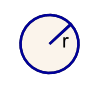Circle Area = π × r2 Circumference = 2 × π × r r = radiusEllipse Area = πab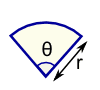Sector Area = ½ × r2 × θ r = radius θ = angle in radians
 Note: h is at right angles to b:### Example: What is the area of this rectangle?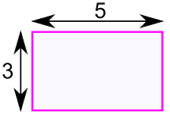The formula is:

Area = w × h
w = width
h = height

We know w = 5 and h = 3, so:

Area = 5 × 3 = 15

### Example: What is the area of this circle?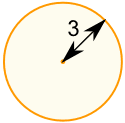Area = π × r2 = π × 32 = π × (3 × 3) = 3.14159... × 9 = 28.27 (to 2 decimal places)

### Example: What is the area of this triangle?Height = h = 12

Base = b = 20

Area = ½ × b × h = ½ × 20 × 12 = 120

A harder example:

### How much does Sam earn cutting this area: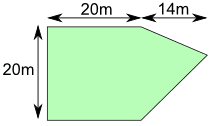Let's break the area into two parts: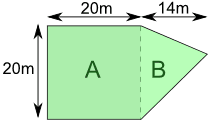Part A is a square:

Area of A = a2 = 20m × 20m = 400m2

Part B is a triangle. Viewed sideways it has a base of 20m and a height of 14m.

Area of B = ½b × h = ½ × 20m × 14m = 140m2

So the total area is:

Area = Area of A + Area of B = 400m2 + 140m2 = 540m2

Sam earns \$0.10 per square meter

Sam earns = \$0.10 × 540m2 = \$54